## The Odds ratio

• The odds ratio is a very useful device for the analysis of categorical data.
• It measures association
• and underlies the maths behind loglinear models and logistic regression.

• What are odds?

• The odds of outcome 1 versus outcome 2 are the probability (or frequency) of outcome 1 divided by the probability (or frequency) of outcome 2.

• Contrast this with probability or proportions: one category's probability is its frequency divided by the total frequency: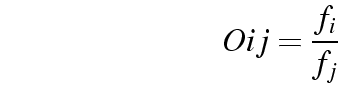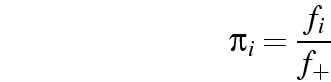• Where there are only two outcomes (e.g., winning and losing):• A horse given odds of 5:2 has 2 chances in 7 (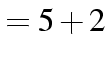) of winning (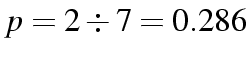).

• Odds measure the frequency or probability of one outcome relative to another.

• Odds ratios involve comparing the odds of a pair of outcomes on one variable, for a pair of categories of a second variable: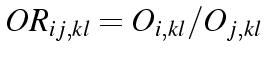Owners Private Total renters Cons 1977 211 2188 Lab 2353 378 2731 Total 4330 589 4919

• The overall odds of owning versus renting are. That is, there are far more owners.

• However, separately for Conservative and Labour voters:

 Conservative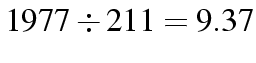Labour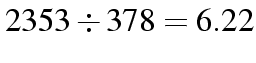Ratio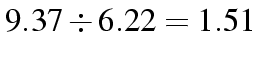• The Odds-Ratio for conservative versus labour voters being owners versus private renters is 1.51. That is, even though labour voters are predominantly house-owners, conservative voters are much more likely to be house-owners.

• A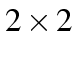table under independence will have an odds ratio of 1, whatever the marginals.

• If we know the odds ratio and the marginals for atable we can calculate all the cell sizes.

• For bigger 2-D tables, we can reconstruct all the cell counts from the marginals and a set of odds ratios that involve all the cells (is sufficient).

• The odds ratios are therefore equivalent to the structure of association.

• Loglinear models essentially define a pattern of odds ratios, apply the marginals to them, and compare the resulting table with the observed table, in pretty much the same way we apply the Pearson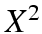test for association. The big difference is the pattern we define can be much more complicated than independence.© Brendan Halpin (e-mail) 23-Apr-2012 Department of Sociology, University of Limerick Taught programme: MA in Sociology (Applied Social Research), Short course, May 14/15 2012: Categorical Data Analysis for Social Scientists